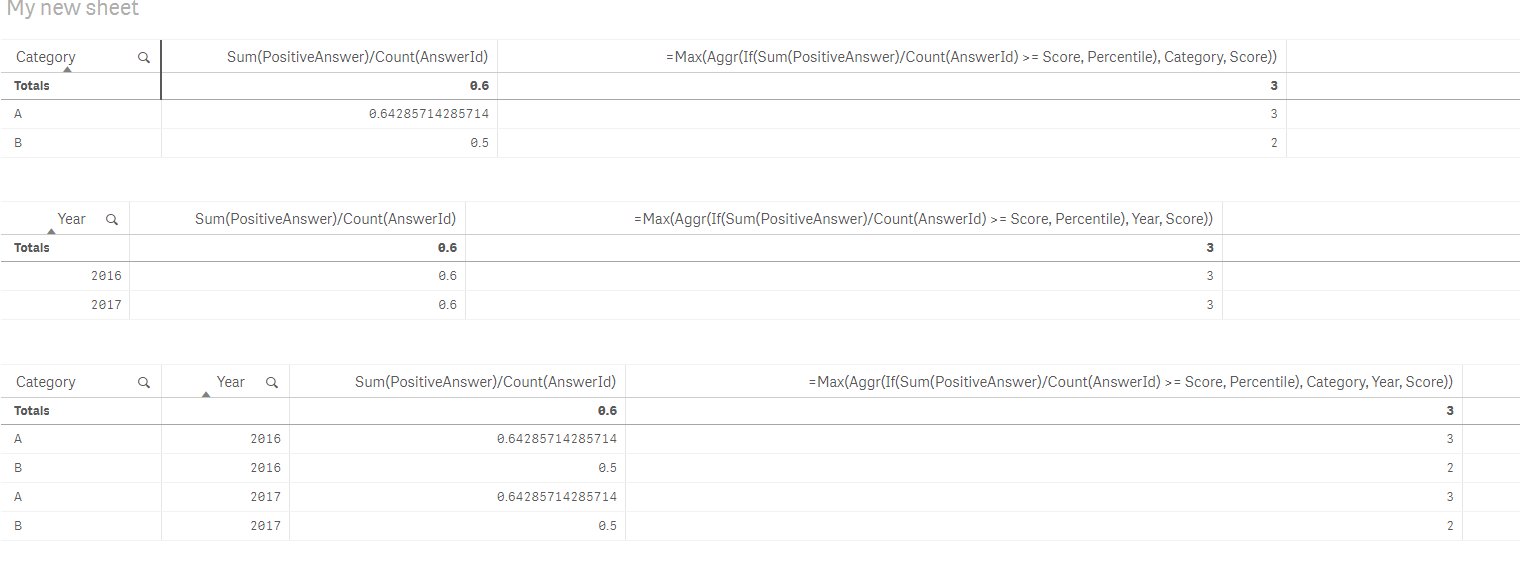# Qlik Sense App DevelopmentNot applicable

## lookup value from a different table that has no joins

In a chart, I'm trying to find any way to either

1) lookup the Percentile range where a calculated aggregate score falls in a table that has a list of Percentiles and the Scores to be compared to.  I want the max percentile where the calculated score is < the percentile score from the table.  See sample data below

or 2) since the calculated score is displayed in a bar chart is there any way to use for example the Above(<score formula>, 0) or something like the Column function in the formula

The primary problem is when I have a working formula, the calculated score is only calculated once per chart rather than for each dimension.  Each dimension is already displaying the calculated score which is why I was hoping something like Above(<score formula>, 0) could work but I haven't had any success.

Here is some very simplified data.  As an example, the calculated score would be something like Sum(PositiveAnswer)/Count(AnswerId).  That measure would display in a bar chart and then we need another measure showing the Percentile it falls in.  So the calculated score needs to be compared to the Score in the Percentiles table to get the Max(Percentile) where the calculated score is less than the Percentile score.

I've been at this for days!  Any help or suggestions is greatly appreciated.

[Percentiles]:
[Percentile, Score
1,0.1
2,0.5
3,0.6
4,0.8
5,0.9];

1,1,2016,A
2,0,2016,A
3,1,2016,A
4,1,2016,A
5,1,2016,A
6,1,2016,A
7,0,2016,A
8,0,2016,A
9,0,2016,A
10,1,2016,A
11,1,2016,A
12,0,2016,A
13,1,2016,A
14,1,2016,B
15,1,2016,B
16,1,2016,B
17,0,2016,B
18,0,2016,B
19,0,2016,B
20,1,2016,A
21,1,2017,A
22,0,2017,A
23,1,2017,A
24,1,2017,A
25,1,2017,A
26,1,2017,A
27,0,2017,A
28,0,2017,A
29,0,2017,A
30,1,2017,A
31,1,2017,A
32,0,2017,A
33,1,2017,A
34,1,2017,B
35,1,2017,B
36,1,2017,B
37,0,2017,B
38,0,2017,B
39,0,2017,B
40,1,2017,A];

1 Solution

Accepted SolutionsMVP

## Re: lookup value from a different table that has no joins

May be this(assuming Category is your dimension)

or this (assuming Year is your dimension)

or this (assuming Category and Year as dimensions)2 RepliesMVP

## Re: lookup value from a different table that has no joins

May be this(assuming Category is your dimension)

or this (assuming Year is your dimension)